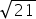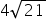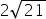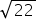Mathematics
Easy

Question

# Suppose the area of a square is 84 sq.cm, find the side length.

##Hint:

## The correct answer is:### We are given the area of a square is 84 sq. cm. We have to find the side length.We have,Area of a square = 84 cm².=> (side)² = 84=> side = √84=> side = 2√21 cm.Hence, the correct option is C.

Area of a rectangle is length × breadth. Since, for a square both length and breadth are equal so its area is (side)².

### Related Questions to study#### With Turito Foundation.#### Get an Expert Advice From Turito.